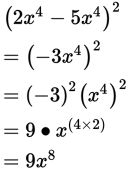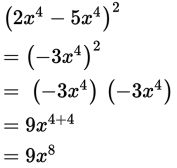# SAT Math Multiple Choice Question 875: Answer and Explanation

### Test Information

Question: 875

5. Which of the following is equivalent to the expression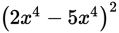?

• A.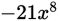• B.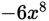• C.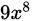• D.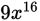Explanation:

C

Difficulty: Easy

Category: Passport to Advanced Math / Exponents

Strategic Advice: When a quantity is squared, don't immediately starting FOILing. Look for like terms that you can combine first. Then follow the rules of exponents or use repeated multiplication to evaluate the power.

Getting to the Answer: If you examine the expression carefully, you'll see that you can combine the two terms inside the parentheses and then square the result:

 Rules of Exponents Repeated Multiplication